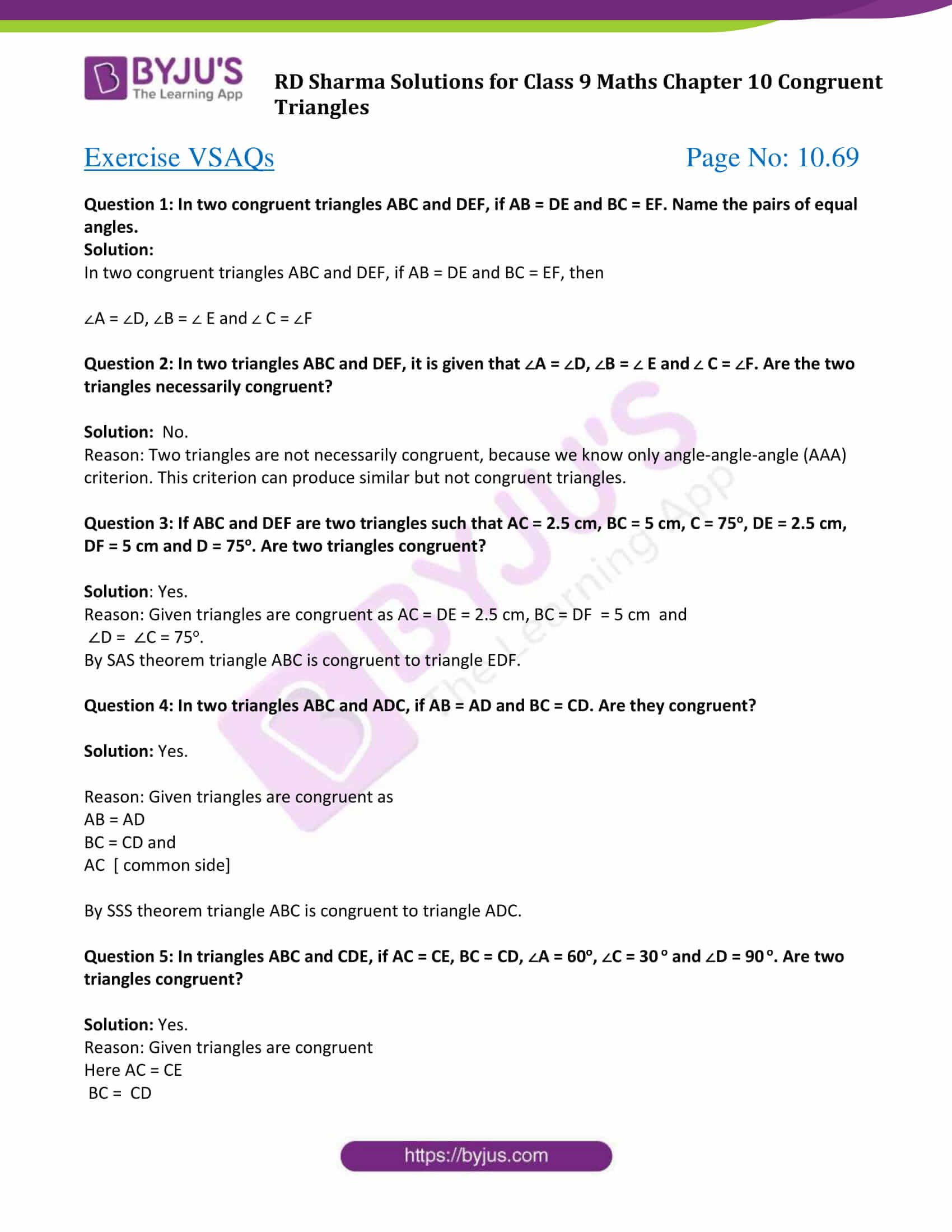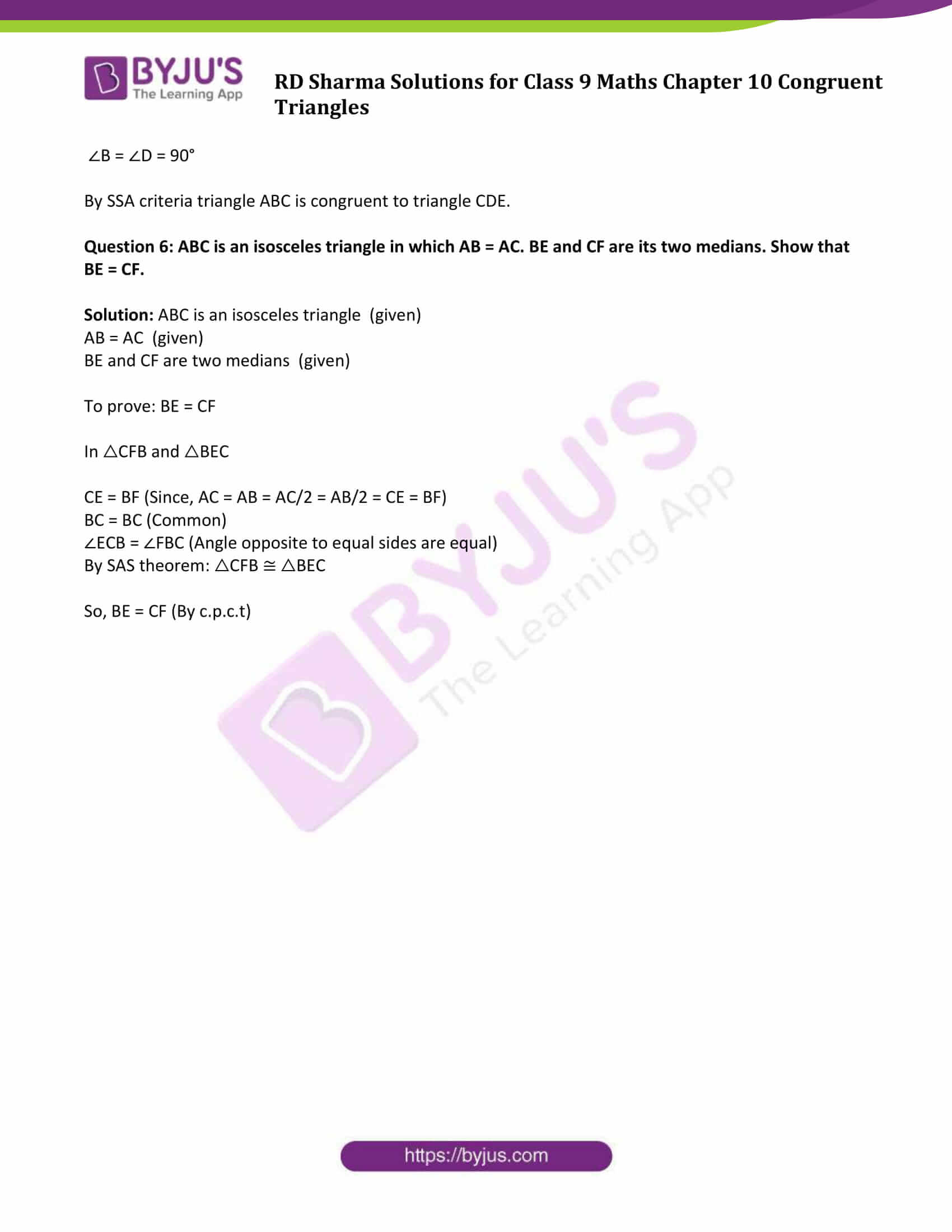# RD Sharma Solutions for Class 9 Maths Chapter 10 Congruent Triangles Exercise VSAQs

RD Sharma Class 9 Mathematics chapter 10 exercise VSAQs Congruent Triangles solutions are provided here. This exercise is specially designed for the revision purpose. These examples would help students to have a better understanding of Congruent Triangles in Class 9 RD Sharma. Students can practice all the facts they have learnt in this chapter and get ready for the exams. Get detailed RD Sharma class 9 solutions from the below link.

## Download PDF of RD Sharma Solutions for Class 9 Maths Chapter 10 Congruent Triangles Exercise VSAQs### Access Answers to Maths RD Sharma Solutions for Class 9 Chapter 10 Congruent Triangles Exercise VSAQs Page number 10.69

Question 1: In two congruent triangles ABC and DEF, if AB = DE and BC = EF. Name the pairs of equal angles.

Solution:

In two congruent triangles ABC and DEF, if AB = DE and BC = EF, then

∠A = ∠D, ∠B = ∠ E and ∠ C = ∠F

Question 2: In two triangles ABC and DEF, it is given that ∠A = ∠D, ∠B = ∠ E and ∠ C = ∠F. Are the two triangles necessarily congruent?

Solution:
No.

Reason: Two triangles are not necessarily congruent, because we know only angle-angle-angle (AAA) criterion. This criterion can produce similar but not congruent triangles.

Question 3: If ABC and DEF are two triangles such that AC = 2.5 cm, BC = 5 cm, C = 75o, DE = 2.5 cm, DF = 5 cm and D = 75o. Are two triangles congruent?

Solution: Yes.

Reason: Given triangles are congruent as AC = DE = 2.5 cm, BC = DF = 5 cm and

∠D = ∠C = 75o.

By SAS theorem triangle ABC is congruent to triangle EDF.

Question 4: In two triangles ABC and ADC, if AB = AD and BC = CD. Are they congruent?

Solution: Yes.

Reason: Given triangles are congruent as

BC = CD and

AC [ common side]

By SSS theorem triangle ABC is congruent to triangle ADC.

Question 5: In triangles ABC and CDE, if AC = CE, BC = CD, ∠A = 60o, ∠C = 30 o and ∠D = 90 o. Are two triangles congruent?

Solution: Yes.

Reason: Given triangles are congruent

Here AC = CE

BC = CD

∠B = ∠D = 90°

By SSA criteria triangle ABC is congruent to triangle CDE.

Question 6: ABC is an isosceles triangle in which AB = AC. BE and CF are its two medians. Show that BE = CF.

Solution: ABC is an isosceles triangle (given)

AB = AC (given)

BE and CF are two medians (given)

To prove: BE = CF

In △CFB and △BEC

CE = BF (Since, AC = AB = AC/2 = AB/2 = CE = BF)

BC = BC (Common)

∠ECB = ∠FBC (Angle opposite to equal sides are equal)

By SAS theorem: △CFB ≅ △BEC

So, BE = CF (By c.p.c.t)

## RD Sharma Solutions for Class 9 Maths Chapter 10 Congruent Triangles Exercise VSAQs

RD Sharma Solutions Class 9 Maths Chapter 10 Congruent Triangles Exercise VSAQs is based on all the concepts covered in this chapter. Students can download this study material now for free and clear all their doubts. After practising this study material, students will be able to classify all geometrical figures on the basis of their shapes.